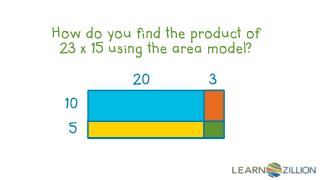DownloadDownload PresentationHow do you find the product of 23 x 15 using the area model?

# How do you find the product of 23 x 15 using the area model?

Télécharger la présentation## How do you find the product of 23 x 15 using the area model?

- - - - - - - - - - - - - - - - - - - - - - - - - - - E N D - - - - - - - - - - - - - - - - - - - - - - - - - - -
##### Presentation Transcript

1. In this lesson you will learn how to multiply multi-digit numbersby using the area model.

2. Product – The result when two numbers are multiplied. For Example: 4 x 5 = 20 product

3. Multiplication is repeated addition. 32 + 32 + 32 + 32 + 32 32 x 5 Multiplication is faster!

4. Multiplication sentences can be represented by using an array. 6 4 4 x 6 = 24

5. Multiplying by powers of 10. 36 x 10 = 360 36 x 100 = 3,600 36 x 1,000 = 36,000

6. Multiplying each digit as if it represents a number of “ones.” It is important to remember the place value of each digit. 36 x 5 = 30 x 5 + 6 x 5

7. Multiply 23 x 15 using the area model. 20 + 3 10 + 5 200 100 30 + 15 445 x 20 3 10 200 30 15 5 100

8. Multiply 225 x 12 using the area model. 2000 400 200 40 50 + 10 2700 200 + 20+ 5 10 + 2 x 200 20 5 200 2000 50 10 400 40 10 2

9. In this lesson you have learned how to multiply multi-digit numbersby using the area model.

10. Multiply 352 x 32 using the area model.

11. Roll a dice 4 times to fill in the blanks and create a multiplication problem like the one below. x • Use the area model to find the product.

12. 1). Find the multiplication sentence that matches the model below. 40 7 • 24 x 37 • 47 x 23 • 47 + 23 • 60 x 10 20 3

13. 2) Multiply 351 x 62 using an area model.